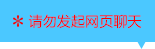| | |

||
APP端下载AndroidiPhone
￥260

|
1011人点赞
568785人已学习
|

3天无理由退款

3天无理由退款：退款将以超级币形式退至您的超级课堂学习账户，便于您重新选购其他课程。恶意退款将被冻结账号。

• 1、对数函数的概念，判断对数函数要注意三点：(1)底数$a$为大于$0$且不为$1$的常数。(2)真数位置上只能有$x$这一项。(3)整个对数式的系数必须是$1$，且后面不能有不为零的常数。对数函数的定义域为$(0,＋\infty )$。确定底数$a$可以采用待定系数法，但不能带入$(1,0)$，因为对数函数的图象一定经过点$(1,0)$
2、 $0＜a＜1$时，图象下降，函数递减。当$a＞1$时，图象上升，函数递增。
3、 图象都在$y$轴右侧，$y$轴是它们的渐近线，值域为$R$。且都经过$(1,0)$这个定点。若两个对数函数的底数互为倒数。它们的图象总关于$x$轴对称。
4、 对比指数函数的图像，它们的定义域和值域恰好相反。都过定点。且单调性受$a$影响的规律是一致的。即底数$0＜a＜1$,两种函数都是单减的；$a＞1$都是单增的。
• 1、两类绝对值函数的图象及常用规律
2、 一种是套$x$的类型，图象左右对称。根据$a$的范围，分为喇叭口朝下和朝上两种图象。常见的还有它的平移变换后的函数，变换依旧遵循“左加右减”原则。对称轴为$x=-k$
3、 第二种是整体套类型，图象都为$v$型，顶点都为$(1,0)$。但要注意$a$会影响每段图象对应的解析式，在画图时，不妨也写出每段的解析式
4、 两种套法相结合的绝对值函数，作图象时，按前面两种画法分步操作即可。图象为关于$x=-k$对称的断开的“$vv$”型，两个顶点在$x$轴上，分别为$(-1-k,0)$和$(1-k,0)$
• 1、一种是由单调性求字母范围。由函数递减推出$0＜a＜1$，由函数递增推出$a＞1$。涉及分段函数单调性满足的条件，以及通过图像判断字母参数的范围，要注意结对数函数的应用—对数函数的单调性与值域合二次函数的知识
2、 第二种是值域相关的题目。当底数固定时，根据单调性，结合图象就能求出相应区间内的值域；当底数有未知字母时，若条件给出的是最值之和，则不需要准确知道哪个是最大值，哪个是最小值，故不需要分类讨论。若给出的是最值之差，或倍数关系，则需要搞清哪个是最大值，哪个是最小值，此时需要按底数分类讨论
• 1、常见的对数不等式有三类
2、 对于第一类对数不等式，解法是将常数$b$化为以$a$为底数的对数$log_{a}a^{b}$，再根据对数函数$y=log_{a}x$的单调性来得到真数$f(x)$与$a^{b}$的大小关系，同时要注意真数$f(x)＞0$
3、 对于第二类对数不等式。根据底数决定的外层对数函数的单调性，得到内层函数$f(x)$与$g(x)$的大小关系。不过要注意，真数都是要大于$0$的。所以我们一般会得到三个不等式组成的不等式组
4、 如果不等式两侧底数不同，就要进行化同底。化同底采用的基本原理一般是换底公式的推论$1$。如果两个对数的底数不能直接相互转化，就要把它们的底数转化成另外同一个数
5、 如果对数式的底数含有参数，就要分类讨论。最后一道题要注意的是，在通过不等式组求交集时，要利用底数分类的前提条件
• 1、讲解两种解对数不等式的高级方法：换元法与图象法
2、 对于第三类对数不等式，及可以化为这种形式的不等式，可以采用是换元法
3、 通过两边同时平方，化同底，取对数等处理，某些不等式都能变成第三类对数不等式的基本形式。注意，在通过$t$的范围求$x$的范围时，真数$x$要满足大于$0$的前提
4、 对于含$x$的项较多，且无法用换元法化简的对数不等式，要尝试用图象法去解决。把含对数式的项和其他类型的项分别放在不等号两边。观察出它们属于那种函数结构，通过图像的上下关系，来求不等式的解集
• 1、利用构造函数法，解决不规则的指数不等式、对数不等式
2、 可以通过移项，使不等号两侧结构相同，根据相似结构构造函数
3、 也可以通过每一项都除以同一个指数式，整体构造出一个单调性确定的函数
4、 对于更加不规则的对数不等式，需要通过换元，恒等式等数学工具进行转化
• 1、主要内容就是利用构造函数法，解决不规则的指数不等式、对数不等式
2、 可以通过移项，使不等号两侧结构相同，根据相似结构构造函数
3、 也可以通过每一项都除以同一个指数式，整体构造出一个单调性确定的函数
4、 对于更加不规则的对数不等式，需要通过换元，恒等式等数学工具进行转化。最后一道题就是一个很典型的例子，同学们要好好揣摩这两大技巧和思维步骤
• 1、利用图像法和换底法解决第二类—底数不同，真数相同的对数式大小比较问题
2、 图像法的关键，是要记住底数和图像位置关系的规律。通过这个规律就能画出底数不同的对数函数图像的大致位置关系，再用一根代表相同真数的竖线，根据交点的上下关系，就能判断出对数值的大小关系。当然，也能反用，通过图像，判断底数的大小关系
3、 换底法，即把题目化为以同底数对数为分母的倒数的大小比较问题
• 1、处理底数、真数都不同的对数式的大小比较的两种方法是标准值法和图像法
2、 对于标准值法，可以选取$0$或$\pm 1$作为标准值。同时要记住一个常用规律：如果底数与真数同大于$1$或同小于$1$，那么对数值大于$0$；如果底数与真数一个大于$1$，一个小于$1$，那么对数值小于$0$
3、 对于图像法，在同一坐标系中画出每个函数的图像，然后找真数对应的点，通过点的高低判断大小关系
• 1、对数型复合函数求定义域依然遵行“由外向内”的原则。对于外层为对数函数的类型，首先要保证真数$f(x)$大于$0$，然后再考虑内层函数$f(x)$的定义域。切忌随意合并原函数，否则会改变定义域。对于内层为对数函数的类型，首先考虑外层函数$y=f(u)$的定义域，求出$u$的范围，即$log_{a}x$的范围，再求出$x$的范围，得到定义域
2、 过定点的问题也非常简单，对于外层为对数函数的复合函数。若$f(m)=1$，则图象过定点$(m,0)$。对于内层为指数函数的复合函数，图象过定点$(1,f(0))$
• 1、对数型复合函数的单调性依然遵循“同增异减”的原则，千万不要忘记真数大于$0$的定义域的潜在限定
2、 对于组合型的对数型复合函数，先尝试根据组合函数单调性的规律来判断，如果行不通，就要进行合并，但要先求定义域，因为合并会改变原函数定义域
• 1、本节课介绍了外层为对数函数的复合函数，$y=log_{a}f(x)$的值域求解技巧
2、 原理是先在定义域的基础上求出内层函数$y=log_{a}u$的值域，再将它作为外层对数函数的定义域，从而求出$y$的范围，即复合函数值域
3、 然后还介绍了一类常见题型：什么情况下$y=log_{a}f(x)$的定义域为$R$，什么情况下它的值域为$R$。注意，借助图象研究会更直观
• 1、本节视频主要内容是内层为对数函数的复合函数的值域求解技巧
2、 基本方法和普通复合函数求值域的方法一样，结合图像去分析。需要注意使用的技巧主要有：对数式化同底，含参二次函数最值讨论。其中最后一道例题含金量很高，体现了非常严谨的数学思维，同学们要注意体会

• 1墩儿
• 2超级学员346136
• 3超级学员5
• 4超级学员555437
• 5超级学员5
• 6超级学员629423
• 7禁欲小山
• 8超级学员873522
• 9超级学员9568441232030人在学
￥ 240 ￥ 0578498人在学
￥ 240 ￥ 1981213297人在学
￥ 240 ￥ 0578520人在学
￥ 300 ￥ 198

• 0
• 点击分享有好礼
••app端下载关注微信号